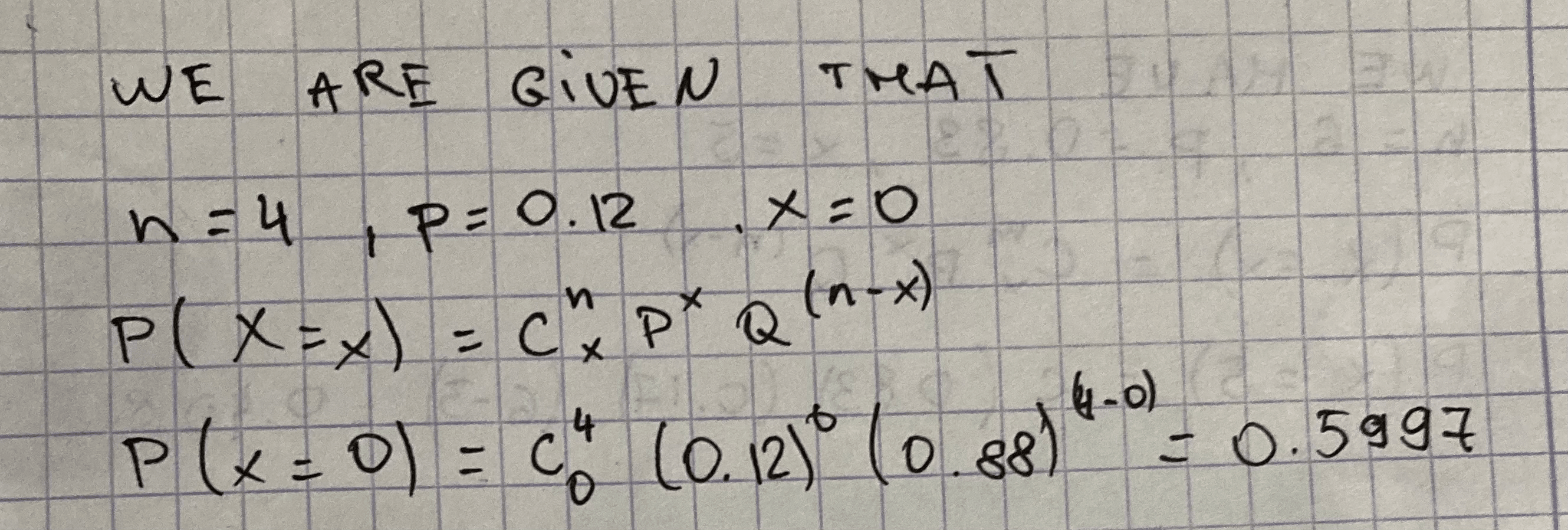Question# Determine the following Binomial Probability: for n=4, p=0.12, x=0.

Binomial probability
ANSWEREDDetermine the following Binomial Probability: for $$n=4, p=0.12, x=0.$$Solution